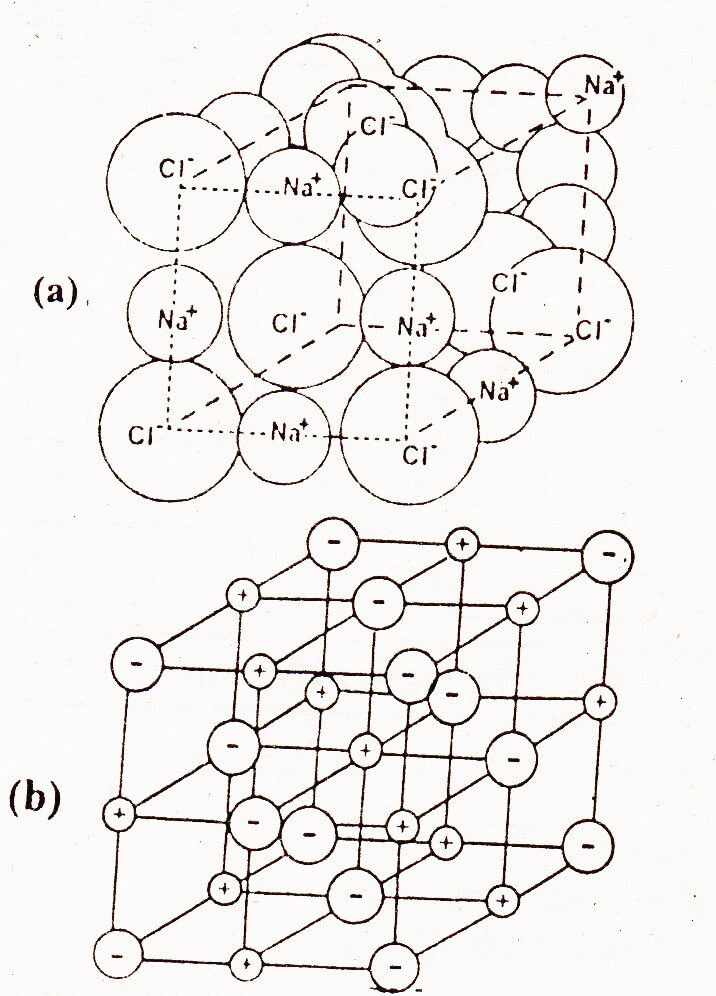Ionic crystals depend upon the structure and the size of their ions. In the structure of NaCl each Na+ ion is surrounded by six chloride ions. The following figure shows how these ions are arranged in the crystal lattice.It is clear that Na+ ion has ten electrons while Cl has 18 electrons. The size of the Cl is bigger than Na+.

The distance between two adjacent ions of the same kind i.e. Cl ions is 5.638. So the distance between two adjacent ions of different kind is 5.63/2 = 2.815Å.

The location of Na+ and Cl is such that each Na+ is surrounded by six Cl ions placed at the corners of a regular octahedron. So the coordination number of six Na+ and Cl ions are not connected to one another by pairs because all six Cl ions are at the same distance away from one Na+ ion. It has been observed that independent molecules of NaCl do exist in the vapour phase.

There are eight Cl ions at the corners of the cube, and each shared amongst eight cubes. Six chloride ions are at the face centers, each shared between two cells. Thus per unit cell there are 6/6 + 6/2 = 4Cl ions. There are equal number of Nations and therefore 4NaCl units are present per unit cell.